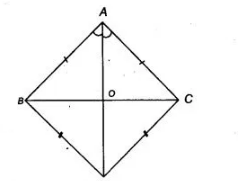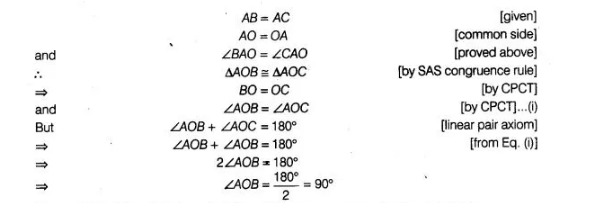# A B C and D B C are two triangles`
Question:

$A B C$ and $D B C$ are two triangles on the same base $B C$ such that $A$ and $D$ lie on the opposite sides of $B C, A B=$ $A C$ and $D B=D C$. Show that $A D$ is the perpendicular bisector of $B C$.

Solution:

Given Two $\triangle A B C$ and $\triangle D B C$ are formed on the same base $B C$ such that $A$ and $D$ lie on the opposite sides of $B C$ such

that $A B=A C$ and $D B=D C$. Also $A D$ intersects $B C$ at $O$. To show $A D$ is the perpendicular bisector of $B C$ i.e., $A D \perp B C$ and

$A D$ bisects $B C$. Proof In $\triangle A B D$ and $\triangle A C D$,

$A B=A C$ [given]

$A D=A D$   [common side]

and  BC=D C  [given]

$\therefore$ $\triangle A B D \cong \triangle A C D$ [by SSS congruence rule]

$\Rightarrow$ $\angle B A D=\angle C A D$ [by CPCT]

i.e., $\angle B A O=\angle C A O$

In $\triangle A O B$ and $\triangle A O C$,Hence, $A D \perp B C$ and $A D$ bisects $B C$ i.e., $A D$ is the perpendicular bisector of $B C$.